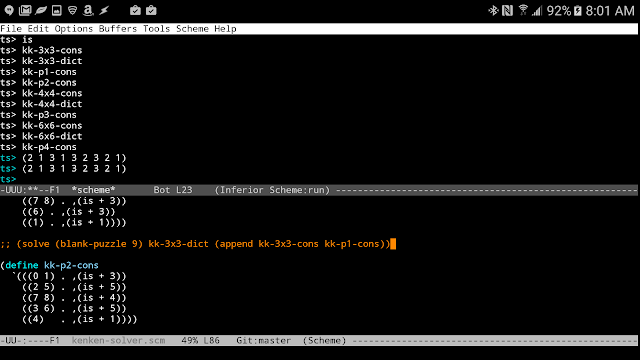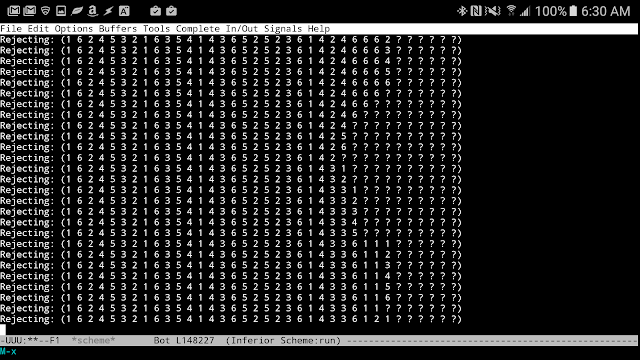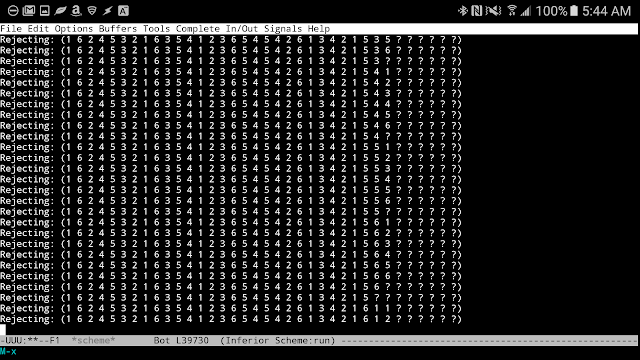## Wednesday, May 18, 2016

### The KenKen Solutiont that Can't

Mark over at brainwagon recounted his frustration with an especially tricky KenKen puzzle and like any good programmer turned to a software solution:

Lately, my lunch hours have been spent working on the NYT Crossword with my lunch companion Tom. While I find that the Thursday crosswords are often beyond my ability to do in the allotted time, between the two of us, more often than not we manage to plow through them. Slowly over time, we’ve begun to solve them slightly quicker, so I’ve branched out to doing the KenKen puzzles.
...
By the end up twenty minutes I was annoyed, knew I had made a mistake, but decided to give into the urge to write a program to solve it. How long could it take?

Well Mark, Challenge Accepted.

After reading his post (and without looking at his solution) I decided I needed to create my own KenKen solver. I busted out my Samsung Galaxy Note 5, Perixx keyboard and a new app configuration: Termux + emacs + TinyScheme and went to work.

After a weekend of thinking through a solution, I had my solver written up fairly quickly:

```;; http://brainwagon.org/2016/05/12/kenken-puzzle-solver/

(define (show . words)
(for-each (lambda (w)
(display w)
(display " "))
words)
(newline))

(define (unique? items)
(if (null? items)
#t
(and (not (member (car items) (cdr items)))
(unique? (cdr items)))))

(define (solved? puzzle)
(not (member '? puzzle)))

(define (blank-puzzle n)
(if (= 0 n)
'()
(cons '? (blank-puzzle (- n 1)))))

(define (try sym puzzle)
(if (null? puzzle)
(error (format "Whoa, can't try ~a in a blank puzzle" sym))
(if (eq? (car puzzle) '?)
(cons sym (cdr puzzle))
(cons (car puzzle)
(try sym (cdr puzzle))))))

(define (ok? puzzle constraints)
(if (null? constraints)
#t
(let* ((params (map (lambda (i) (list-ref puzzle i))
(car (car constraints))))
(valid? (if (solved? params)
(apply (cdr (car constraints)) params)
#t)))
(and valid? (ok? puzzle (cdr constraints))))))

(define (solve puzzle dictionary constraints)
(define (go puzzle pool)
(cond ((solved? puzzle)
puzzle)
((null? pool) #f)
(else
(let* ((next-attempt (try (car pool) puzzle))
(valid (and (ok? next-attempt constraints)
(go next-attempt dictionary))))
(if valid
valid
(begin
;; (show "Rejecting:" next-attempt)
(go puzzle (cdr pool))))))))
(go puzzle dictionary))

(define (uni . args)
(unique? args))

(define (is op val)
(lambda args
(or (= val (apply op args))
(= val (apply op (reverse args))))))
```

The program works by taking in a blank puzzle, a dictionary of values to solve with (in a 4x4 puzzle, that would be (1 2 3 4)) and a set of constraints and does a brute force attack to find a combination of symbols that satisfy all the constraints. KenKen puzzles assume that all rows and columns are unique and that clusters of cells either add, subtract, multiply or divide to form a particular value; these form my constraints.

Here's the code to solve a 3x3 puzzle:

```(define kk-3x3-cons
`(((0 1 2) . ,uni)
((3 4 5) . ,uni)
((6 7 8) . ,uni)
((0 3 6) . ,uni)
((1 4 7) . ,uni)
((2 5 8) . ,uni)))

(define kk-p1-cons
`(((0 3) . ,(is + 3))
((2 5 4) . ,(is + 8))
((7 8) . ,(is + 3))
((6) . ,(is + 3))
((1) . ,(is + 1))))

(define kk-3x3-dict '(1 2 3))

(solve (blank-puzzle 9) kk-3x3-dict (append kk-3x3-cons kk-p1-cons))
```

For clarity, the first set of constraints ensure that every row and column is unique, whereas the second set of constraints describe this particular puzzle. For example, grid entries 2, 5 and 4 must add up to 8.

One compromise I made was to have the solver work in terms of a list rather than a grid. The result was that I had to create little paper maps to help me translate from grid to list coordinates:For 3x3 and 4x4 puzzles my little solution worked. In fact, I spent more time debugging typos in my transcription of constraints than I did in writing the actual core solution. Here's some proof that this actually runs on my cell phone:Unfortunately, my brute-force solution just doesn't cut it for the 6x6 puzzle Mark was working on. I setup the constraints and kicked the program off:

```
(define kk-6x6-cons
`(((00 01 02 03 04 05) . ,uni)
((06 07 08 09 10 11) . ,uni)
((12 13 14 15 16 17) . ,uni)
((18 19 20 21 22 23) . ,uni)
((24 25 26 27 28 29) . ,uni)
((30 31 32 33 34 35) . ,uni)
((00 06 12 18 24 30) . ,uni)
((01 07 13 19 25 31) . ,uni)
((02 08 14 20 26 32) . ,uni)
((03 09 15 21 27 33) . ,uni)
((04 10 16 22 28 34) . ,uni)
((05 11 17 23 29 35) . ,uni)))

(define kk-6x6-dict '(1 2 3 4 5 6))

(define kk-p4-cons
`(((0 1 6) . ,(is * 12))
((2 3 4) . ,(is + 11))
((5 11)    . ,(is + 7))
((17 23 29) . ,(is + 8))
((28 34 35) . ,(is * 72))
((27 33) . ,(is + 5))
((32) . ,(is + 3))
((30 31) . ,(is - 1))
((12 18 24 25) . ,(is + 12))
((13 19) . ,(is / 2))
((20 26) . ,(is / 2))
((14 15 16 10) . ,(is + 19))
((9) . ,(is + 3))
((7 8) . ,(is - 5))
((21 22) . ,(is - 5))))

(solve (blank-puzzle 36) kk-6x6-dict (append kk-p4-cons kk-6x6-cons))
```

I enabled some debugging and got a whole lot of this:After hours of waiting for a solution to be spit out, I finally killed the process. Would it eventually complete and give me the answer? Did I have a bug in my constraints or program that insures it won't even give me an answer? I don't know. But for now, I need to put this problem down and return to it when I'm ready to try a new approach.

Mark, my hat is off to you for your 27 minute solution that completes in 1 millisecond! You da man!

While I don't have my solution yet, this was hardly a bust. A few positive notes:

• A brute force recursive solution was surprisingly easy to write for this sort of problem, and for small enough problem sets, works well. You essentially leverage the interpreter to gain access to backtracking, rather than needing to build it out explicitly.
• Scheme sexpr's make an ideal 'UI' to this sort of problem, with the constraints being comparably easy to read and write without any additional coding effort.
• Termux is sweet. I'm not sure it's going to replace GNURoot, but it's certainly a top notch option. The API opens up some impressive scripting options I'm going to have to explore in another post.
• TinyScheme is a beast. I threw this massive recursive problem at it and it just chugged away. I let the program spin for hours, and it didn't bat an eye. I suppose that's what Tail Call Optimization is all about, but still, it's remarkable to see it in action.
• The solution I came up with is nice and flexible and should work for related puzzles, such as Sudoku. I hope I can keep this general constraint solving nature while also gaining some critical speed.

So yeah, KenKen 1, Ben 0. But this isn't over. I'll be back.